# Auto-Sklearn：通过自动化加速模型开发周期

2021/08/29 10:34## 背景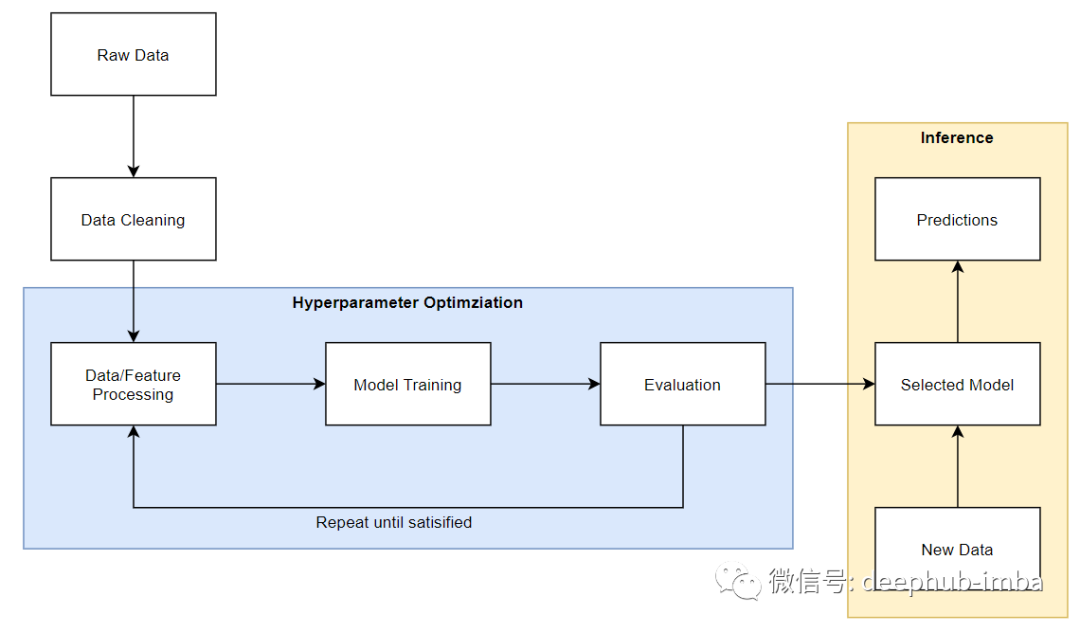## 超参数优化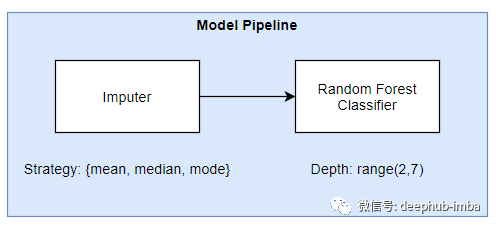Grid Search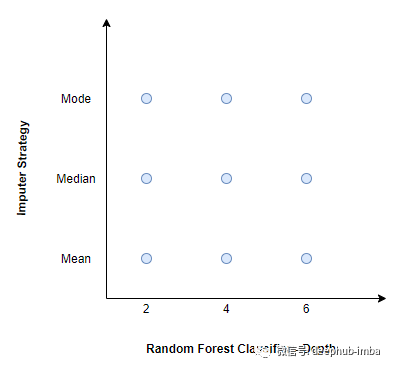Random Search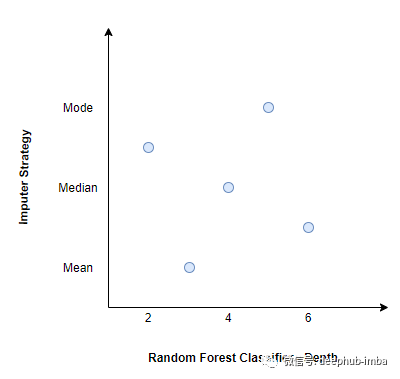Grid和Random Search中的超参数集是相互独立选择的。这两种方法都没有利用以前的训练和评估试验的结果来改善下一次试验的结果。进行超参数优化的一种更有效的方法是利用以前试验的结果来改进下一次试验的超参数选择。这种方法被用于贝叶斯优化。

## Auto-Sklearn

AutoML是对机器学习管道中的数据预处理、特征预处理、超参数优化、模型选择和评估等任务进行自动化的过程。Auto-Sklearn使用流行的Scikit-Learn机器学习框架自动完成上述任务。下面的图片展示了自动学习的工作原理。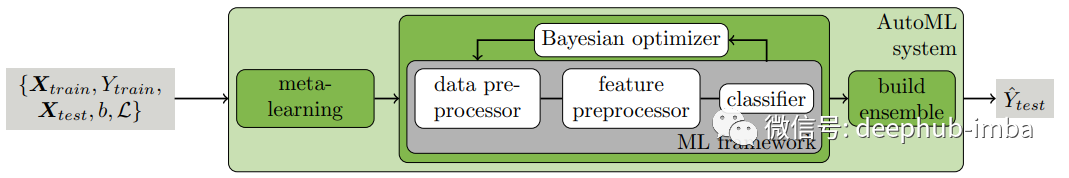Auto-Sklearn使用贝叶斯优化和热启动(元学习)来找到最优的模型管道，并在最后从单个模型管道构建一个集成。让我们检查Auto-Sklearn框架中的不同组件。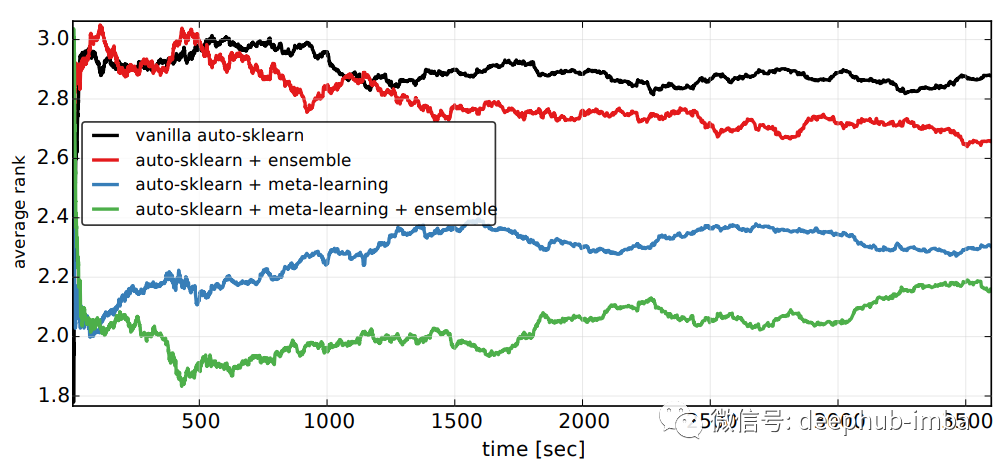Auto-Sklearn对数据的预处理顺序如下:。

1. 分类特征独热编码

2. 使用平均数、中位数或模式的归因

3. 归一化

4. 使用类权重平衡数据集

1. 利用主成分分析、SCV、核主成分分析或ICA进行矩阵分解

2. 单变量特征选择

3. 基于分类特征选择

4. 特征聚类

5. 核逼近（Kernel approximations）

6. 多项式特征扩展

7. 特征嵌入

8. 稀疏表示与变换

## 代码实例

pip install auto-sklearn==0.13

import pandas as pdimport sklearn.metricsfrom sklearn.model_selection import train_test_split, StratifiedKFoldfrom autosklearn.classification import AutoSklearnClassifierfrom autosklearn.metrics import (accuracy,                                 f1,                                 roc_auc,                                 precision,                                 average_precision,                                 recall,                                 log_loss)

df = pd.read_csv('bank-additional-full.csv', sep = ';')

Auto-Sklearn要求列都是数字的，所以让我们现在转换它。

num_cols = ['ge', 'duration', 'campaign', 'pdays', 'previous', 'emp.var.rate', 'cons.price.idx', 'cons.conf.idx', 'euribor3m', 'nr.employed']cat_cols = ['job', 'marital', 'education', 'default', 'housing', 'loan', 'contact', 'month', 'day_of_week', 'poutcome']df[num_cols] = df[num_cols].apply(pd.to_numeric)df[cat_cols] = df[cat_cols].apply(pd.Categorical)y = df.pop('y')X = df.copy()X_train, X_test, y_train, y_test = train_test_split(X, y, test_size = 0.2, random_state=1, stratify=y)

skf = StratifiedKFold(n_splits=5)  clf = AutoSklearnClassifier(time_left_for_this_task=600,                            max_models_on_disc=5,                            memory_limit = 10240,                            resampling_strategy=skf,                            ensemble_size = 3,                            metric = average_precision,                            scoring_functions=[roc_auc, average_precision, accuracy, f1, precision, recall, log_loss])

max_models_on_disc:限制要保留的模型数量

memory_limit:希望使用的内存数量(以MB为单位)

Resampling_strategy:交叉验证重采样策略。

ensemble_size:要包含在ensemble中的模型数量。Auto-Sklearn提供了一个选项，在创建单个模型之后，通过采用加权方式获ensemble_size模型数量来创建集成模型。

metric:我们想要优化的度量指标

scoing_function:我们想要评估模型的一个或多个指标

clf.fit(X = X_train, y = y_train)

df_cv_results = pd.DataFrame(clf.cv_results_).sort_values(by = 'mean_test_score', ascending = False)df_cv_results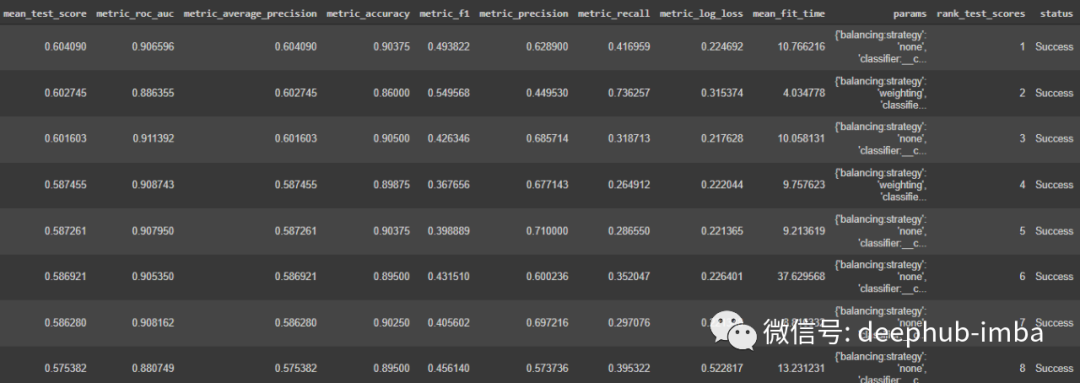clf.leaderboard(detailed = True, ensemble_only=False)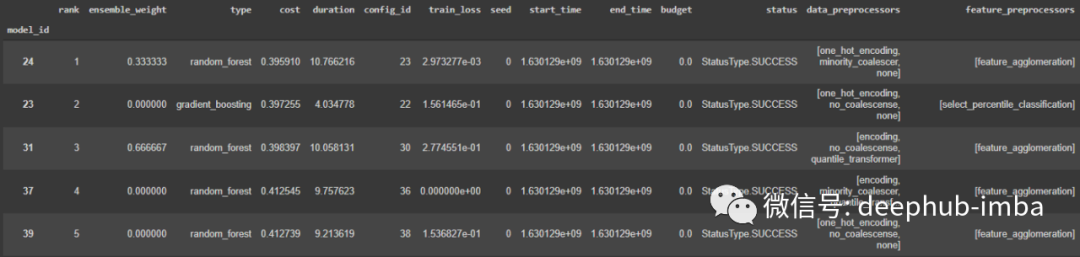clf.get_models_with_weights()

clf.sprint_statistics()

clf.refit(X = X_train, y = y_train)

dump(clf, 'model.joblib')

clf = load('model.joblib')y_probas = clf.predict_proba(X_test)pos_label = 'yes'y_proba = y_probas[:, clf.classes_.tolist().index(pos_label)]

## 引用

 Efficient and Robust Automated Machine Learning

 Supplementary material for Efficient and Robust Automated Machine Learning

 Auto-Sklearn API documentation0 评论
0 收藏
0